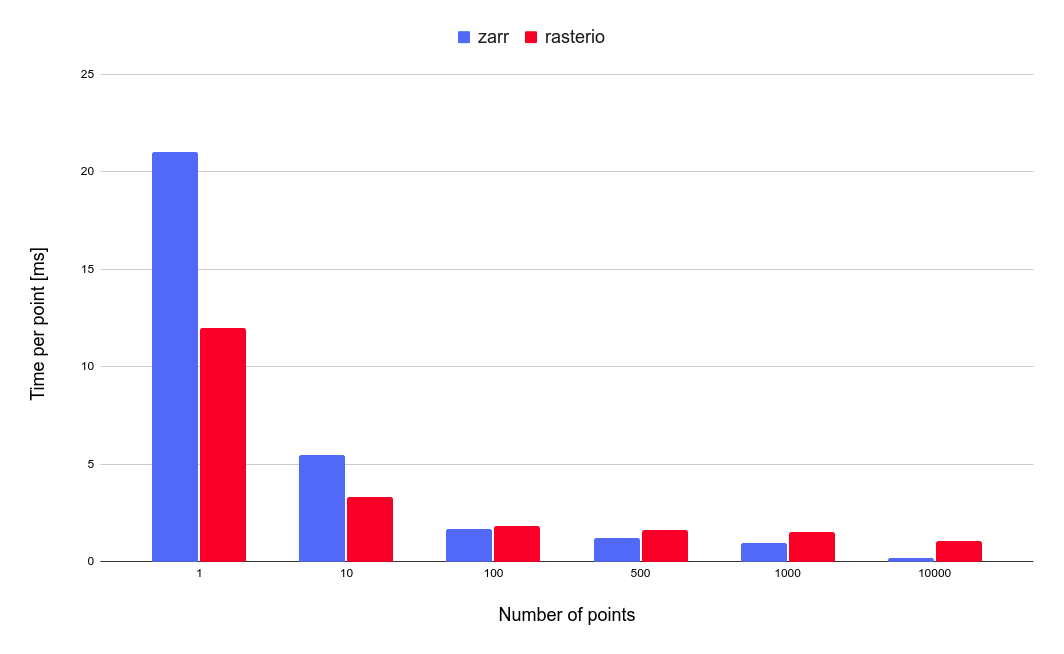# Sampling geotiffs with zarr and tifffile

To sample multiple points from an array in Open Topo Data, I use rasterio something like this:

``````z = []
with rasterio.open(raster_path) as f:
for row, col in points:
window = rasterio.windows.Window(col, row, 1, 1)
indexes=1,
window=window,
)
z.append(z_array)
``````

Rasterio (via gdal) is well tested and supports a huge range of raster formats and several interpolation schemes. But the reading is faster with zarr and tifffile:

``````f = tifffile.imread(path, aszarr=True)
za = zarr.open(f, mode="r")
z = za.get_coordinate_selection((rows, cols))
f.close()
``````

For non-integer rows and cols, you’ll need to do interpolation yourself. Nearest-neighbour interpolation can be done by rounding the rows and cols:

``````f = tifffile.imread(path, aszarr=True)
za = zarr.open(f, mode="r")
rows = np.round(rows).astype(int)
cols = np.round(cols).astype(int)
z = za.get_coordinate_selection((rows, cols))
f.close()
``````

while bilinear interpolation needs a bit more numpy:

``````def bilinear_interpolation(squares, rows, cols):
# Check input shapes.
n_points = len(rows)
assert squares.shape == n_points
assert squares.shape == 2
assert squares.shape == 2

# Use fractional rows/cols to simplify formula.
r = rows % 1
c = cols % 1

# Do interpolation.
f = np.asarray(squares)
bl = np.zeros(n_points, dtype=squares.dtype)
bl += f[:, 0, 0] * (1 - r) * (1 - c)
bl += f[:, 1, 0] * r * (1 - c)
bl += f[:, 0, 1] * (1 - r) * c
bl += f[:, 1, 1] * r * c
return bl

def buffer_indices_to_squares(rows, cols):
r_squares = []
c_squares = []
for row, col in zip(rows, cols):
r = int(row)
c = int(col)
r_squares.append(np.array([[r, r], [r + 1, r + 1]]))
c_squares.append(np.array([[c, c + 1], [c, c + 1]]))
r_squares = np.array(r_squares)
c_squares = np.array(c_squares)
return r_squares, c_squares

r_squares, c_squares = buffer_indices_to_squares(rows, cols)
r_flat = r_squares.flatten()
c_flat = c_squares.flatten()
squares_flat = za.get_coordinate_selection((r_flat, c_flat))
squares = squares_flat.reshape(r_squares.shape)
z = bilinear_interpolation(squares, rows, cols)
f.close()
``````

zarr adds a little latency for single-point samples, but for multiple points performance is much better: I suspect it caches tiff blocks better.